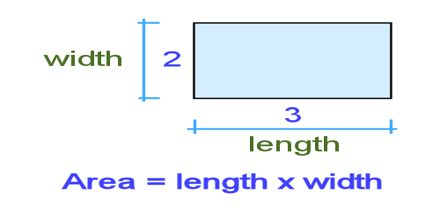Mathematic

# Lecture on Length and AreaThe aim of this lecture is to present on Length and Area. The accuracy of a measurement is how close that measurement is to the true value. This is restricted or limited by the accuracy of the measuring instrument. The ruler is marked in centimetres, so any length measured with it can only be given to the nearest centimetre. Formulas for area and volume are based on lengths. For example, the area of a circle equals π times the square of the length of its radius, and the volume of a rectangular box is the product of its three linear dimensions: length, width, and height.